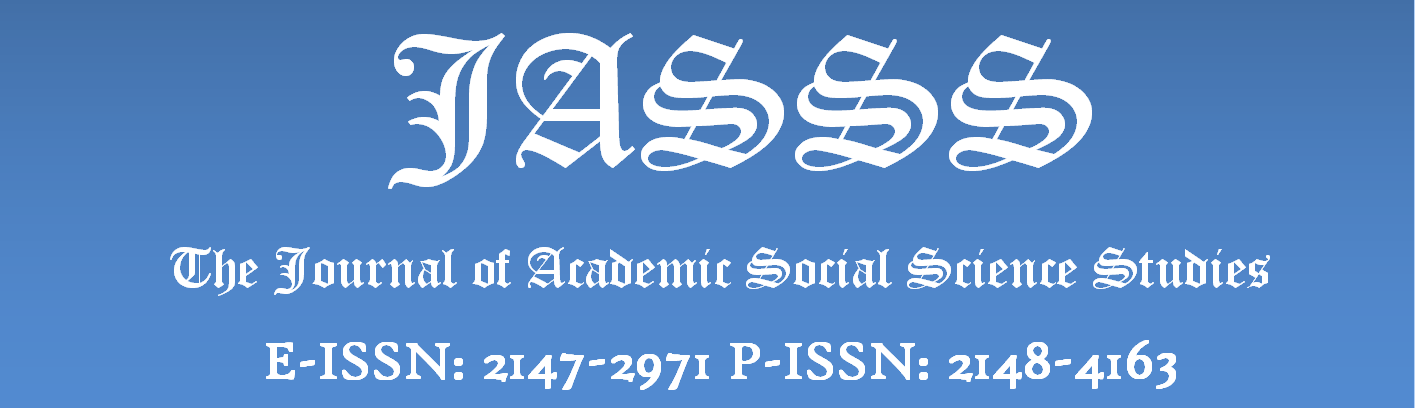### EXAMINATION OF THE MISTAKES MADE BY 7TH-GRADE STUDENTS IN RELATION TO ALGEBRAIC EXPRESSIONS

The aim of this study is to investigate the mistakes made by secondary school 7th-grade students in relation to algebraic expressions. In accordance with this aim, the sample of the study consists of 150 7th-grade students studying at secondary schools in Trabzon city centre in the 2013-2014 academic year. In this study, the case study method was used. In the study, a knowledge test was applied in order to determine the mistakes made by the students in the subject of algebra. The data obtained from in this study were analysed using the content analysis method. From the data obtained from the study, it was observed that the students’ knowledge of algebraic expressions was not at the desired level, and many mistakes were made in the algebra knowledge test applied. Some of these mistakes made are the student’s ignoring the variable, solving the given algebraic expression by transforming it into an equation, using the x variable instead of the variable given in the question, and establishing the equation for the given problem incorrectly

#### Keywords

Algebraic Expressions, Variables, Algebraic Verbal Problems, Student Mistakes, Forming and Solving E

Author : Yasin SOYLU - - Betül ŞİMŞEK
Number of pages: 47-64
DOI: http://dx.doi.org/10.9761/JASSS7806
Full text:The Journal of Academic Social Science Studies